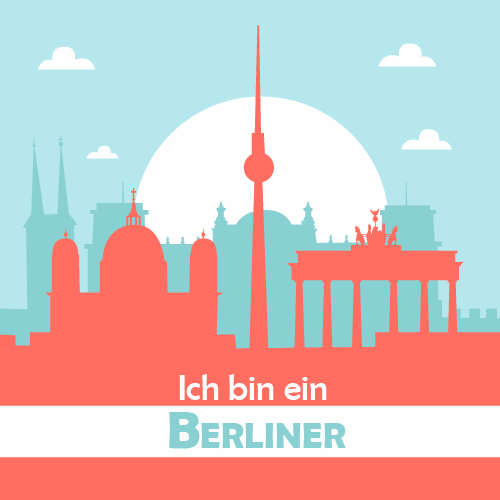## Transcript

Next

• camel
• =
• on

• lion
• =
• on

• snake
• =
• on

• elephant
• =
• on

• monkey
• =
• on

• avancement
• =
• 0

• giraffe
• =
• on

• frog
• =
• on

• puppy
• =
• on

• snake
• =
• on
• 4

• frog
• =
• on
• 6

• giraffe
• =
• on
• 1

• lion
• =
• on
• 2

• elephant
• =
• on
• 0

• puppy
• =
• on
• 7

• camel
• =
• on
• 3

• monkey
• =
• on
• 5

Well done! You have got a puppy!

• avancement
• =
• 8
• 8

To free the elephant, put the correct cage for each animal.

• avancement
• +
• 1

• elephant
• =
• cache

Challenge completed!

Next

To free the giraffe, label the animals correctly

frog

puppy

• avancement
• +
• 1

• giraffe
• =
• cache

giraffe

camel

lion

elephant

monkey

snake

Next

Challenge completed!

To free the lion, work out how many lions there are on this picture.

• avancement
• +
• 1

• lion
• =
• cache

Challenge completed!

Next

Check

To free the camel, you need to match up the animals and their description as they are described in the book "Dear Zoo".

Challenge completed

Next

• camel
• =
• cache

• avancement
• +
• 1

To free the snake, put the magnifiers on the correct animals.
Do you recognise their skins?

• snake
• =
• cache

• avancement
• +
• 1

Challenge completed

Next

To free the puppy, can you spell the words?

l _ _ _

i

o

n

r

g

a

c

m

e

l

p

u

y

To free the puppy, can you spell the words?

l i _ _

i

o

n

r

g

a

c

m

e

l

p

u

y

To free the puppy, can you spell the words?

l i o _

i

o

n

r

g

a

c

m

e

l

p

u

y

Well done!

Next

l i o n

To free the puppy, can you spell the words?

f _ _ _

i

o

n

r

g

a

c

m

e

l

p

u

y

To free the puppy, can you spell the words?

f r _ _

i

o

n

r

g

a

c

m

e

l

p

u

y

To free the puppy, can you spell the words?

f r o _

i

o

n

r

g

a

c

m

e

l

p

u

y

Well done!

Next

f r o g

To free the puppy, can you spell the words?

c _ _ _ _

i

o

n

r

g

a

c

m

e

l

p

u

y

To free the puppy, can you spell the words?

c a _ _ _

i

o

n

r

g

a

c

m

e

l

p

u

y

To free the puppy, can you spell the words?

c a m _ _

i

o

n

r

g

a

c

m

e

l

p

u

y

To free the puppy, can you spell the words?

c a m e _

i

o

n

r

g

a

c

m

e

l

p

u

y

Well done!

Next

c a m e l

To free the puppy, can you spell the words?

p _ _ _ _

i

o

n

r

g

a

c

m

e

l

p

u

y

To free the puppy, can you spell the words?

p u _ _ _

i

o

n

r

g

a

c

m

e

l

p

u

y

To free the puppy, can you spell the words?

p u p _ _

i

o

n

r

g

a

c

m

e

l

p

u

y

To free the puppy, can you spell the words?

p u p p _

i

o

n

r

g

a

c

m

e

l

p

u

y

Well done!

p u p p y

Next

• avancement
• +
• 1

• puppy
• =
• cache

Try again!

Try again

Let's do some animal counting! Which one is more and which is less?

Next

• <
• >
• =

• >
• <
• =

• =
• >
• <

Which one is more and which is less?

• avancement
• +
• 1

• frog
• =
• cache

5 frogs

15 frogs

2 lions

3 lions

2 giraffes and 4 giraffes

3 giraffes and 3 giraffes

Next

• <
• >
• =

• =
• >
• <

• >
• <
• =

one

two

three

four

five

six

seven

eight

How many animals are hiding here?

one

two

three

four

five

six

seven

eight

How many animals are hiding here?

one

two

three

four

five

six

seven

eight

How many animals are hiding here?

one

two

three

four

five

six

seven

eight

How many animals are hiding here?

one

two

three

four

five

six

seven

eight

How many animals are hiding here?

• avancement
• +
• 1

• monkey
• =
• cache